##### Chemistry Workbook For Dummies with Online PracticeLike the difference in their names, the practical difference between molarity and molality is subtle. Take a close look at their definitions, expressed next to one another in the following equations: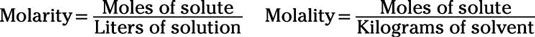The numerators in molarity and molality calculations are identical, but their denominators differ greatly. Molarity deals with liters of solution, while molality deals with kilograms of solvent. A solution is a mixture of solvent and solute; a solvent is the medium into which the solute is mixed.

A further complication to the molarity/molality confusion is how to distinguish between their variables and units. To clarify this plethora of m-words and their abbreviations, check out the table.

M Words Related to Concentration
Name Variable Unit Abbreviation
Molarity M M
Molality m m
Moles -- mol
Occasionally, you may be asked to calculate the mole fraction of a solution, which is the ratio of the number of moles of either solute or solvent in a solution to the total number of moles of solute and solvent in the solution. Chemists defined this quantity using the variable X. Of course, chemists still need to distinguish between the mole fractions of the solute and the solvent, which unfortunately both start with the letter s.

To avoid confusion, they decided to abbreviate solute and solvent as A and B, respectively, in the general formula. In practice, the chemical formulas of the solute and solvent are usually written as subscripts in place of A and B. For example, the mole fraction of sodium chloride in a solution would be written as XNaCl.

In general, the mole ratio of the solute in a solution is expressed as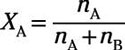where nA is the number of moles of solute and nB is the number of moles of solvent. The mole ratio of the solvent is then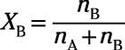.

These mole fractions are useful because they represent the ratio of solute to solution and solvent to solution very well and give you a general understanding of how much of your solution is solute and how much is solvent.

Here's an example: How many grams of dihydrogen sulfide (H2S) must you add to 750 g of water to make a 0.35 m solution?

This problem gives you molality and the mass of a solvent and asks you to solve for the mass of solute. Because molality involves moles and not grams of solute, you first need to solve for moles of solvent, and then you use the gram formula mass of sodium chloride to solve for the number of grams of solute.

Before plugging the numbers into the molality equation, you must also note that the problem has given you the mass of the solvent in grams, but the formula calls for it to be in kilograms. Moving from grams to kilograms is equivalent to moving the decimal point three places to the left. Plugging everything you know into the equation for molality gives you the following: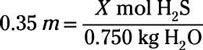Solving for the unknown gives you 0.26 mol of H2S in solution. You then need to multiply this mole value by the molecular mass of H2S to determine the number of grams that need to be added: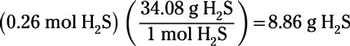So, you require 8.9 grams of dihydrogen sulfide (H2S) to make a 0.35 m solution.